Photonics Research, 2023, 11 (9): 1500, Published Online: Aug. 11, 2023

### All-plasmonic optical leaky-wave antenna with a low sidelobe level

1 School of Electronic Science and Engineering, University of Electronic Science and Technology of China (UESTC)https://ror.org/04qr3zq92, Chengdu 611731, China
2 State Key Laboratory of Terahertz and Millimeter Wave, City University of Hong Kong, Hong Kong 999077, China
3 Department of Electrical Engineering, City University of Hong Kong, Hong Kong 999077, China
Figures & Tables

#### Fig. 1. (a) 3D schematic view of the proposed low-sidelobe plasmonic antenna. (b) Cross-section view of a uniform plasmonic gap waveguide. (c) E-field distribution of the plasmonic gap mode. The parameters are w=350  nm, g=200  nm, and t=100  nm.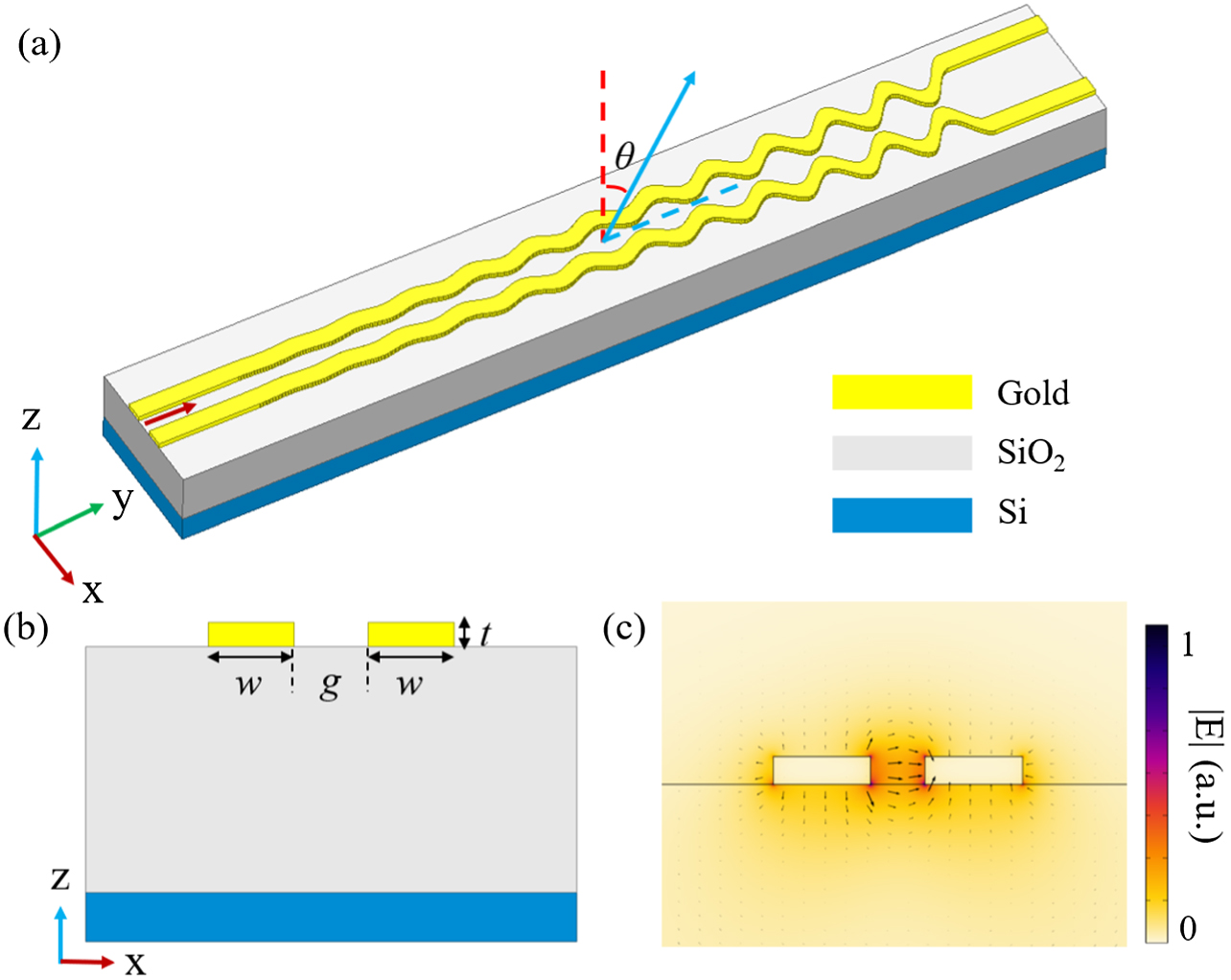#### Fig. 2. Schematic views of (a) uniform plasmonic gap waveguide and (b) sinusoidally modulated antenna. (c) Metal absorption loss αc and normalized phase constant versus gap width g0. (d) Average attenuation constant α of the system and leakage factor αr of the antenna as a function of modulation amplitude g1 with g0=600  nm.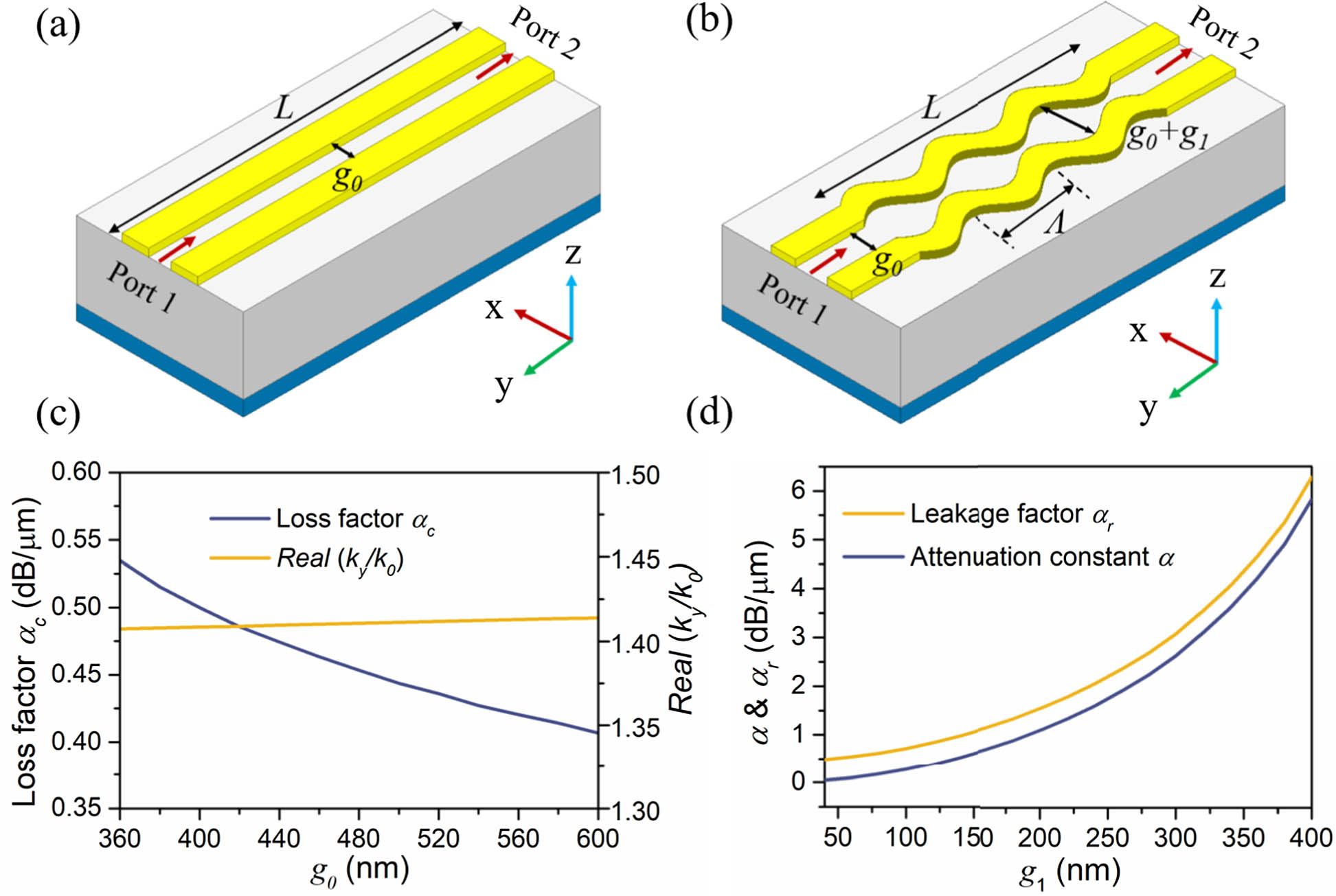#### Fig. 3. (a) Target Chebyshev amplitude distribution along antenna radiation aperture. (b) Theoretical modulation amplitudes and leakage factors for the 12 radiation periods. The blue symbols represent the fitting points with a quadratic function formula. (c) Simulated far-field patterns on the yoz plane at 1500, 1550, and 1600 nm. (d) Radiation efficiency and directivity of the proposed LWA within the wavelength range of 1500 to 1600 nm.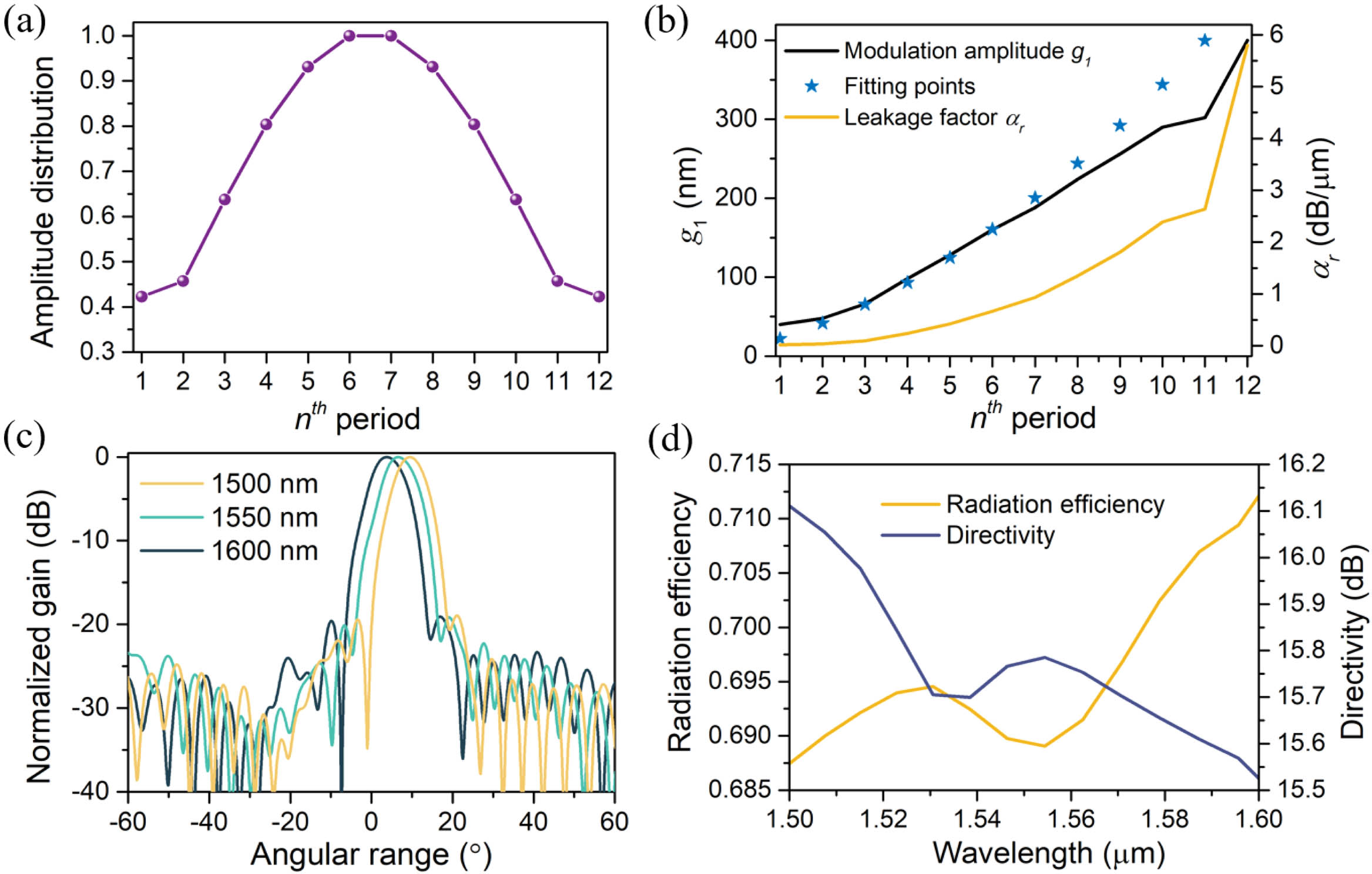#### Fig. 4. (a) Proposed antenna under the coordinate system. (b) 3D radiation pattern of the designed antenna. (c) Radiation patterns in the xoz and yoz planes. (d) Port reflection coefficient S11 as a function of wavelength.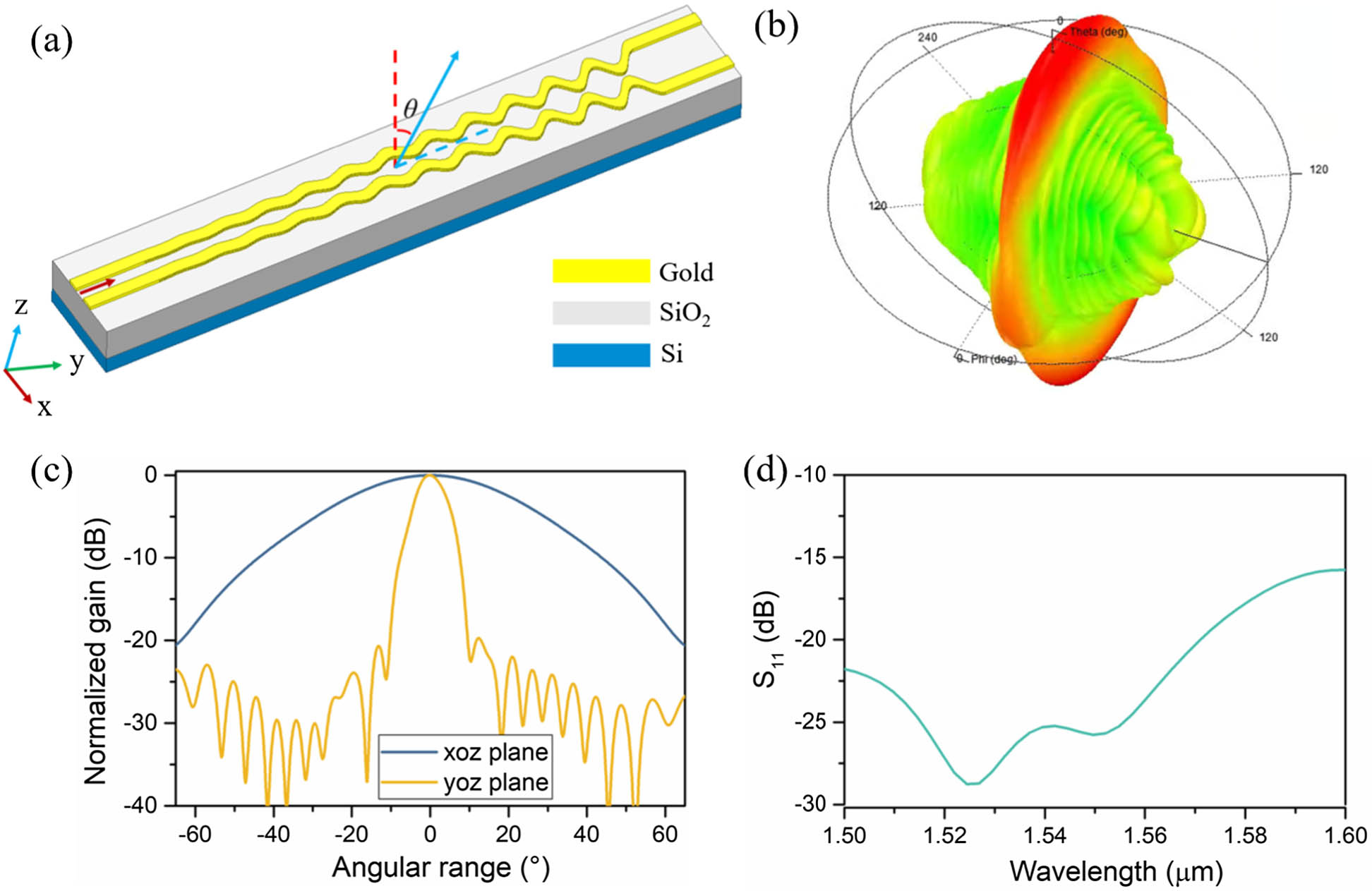#### Fig. 5. SEM image of the (a) referenced structure and (b) fabricated array composed of designed SLL antennas. Measured far-field pattern of the (c) referenced array and (d) proposed design. (e) Measured Fourier-space images of the proposed design at wavelengths of 1527, 1550, and 1570 nm.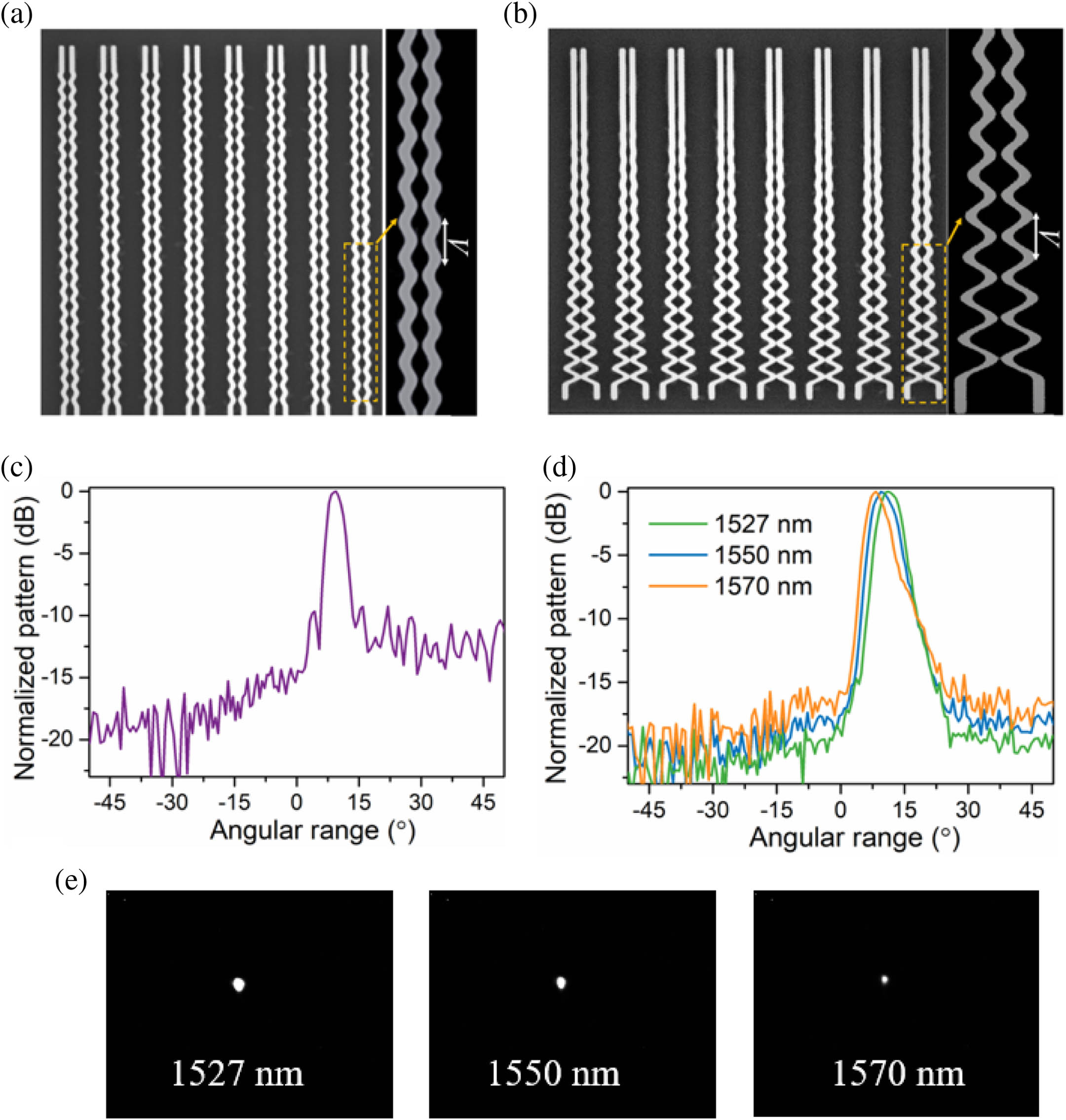#### Fig. 6. (a) Schematic diagram of the operating principle of the proposed leaky-wave antenna. (b) Theoretical normalized radiation pattern in the yoz plane based on the designed Chebyshev amplitude distribution.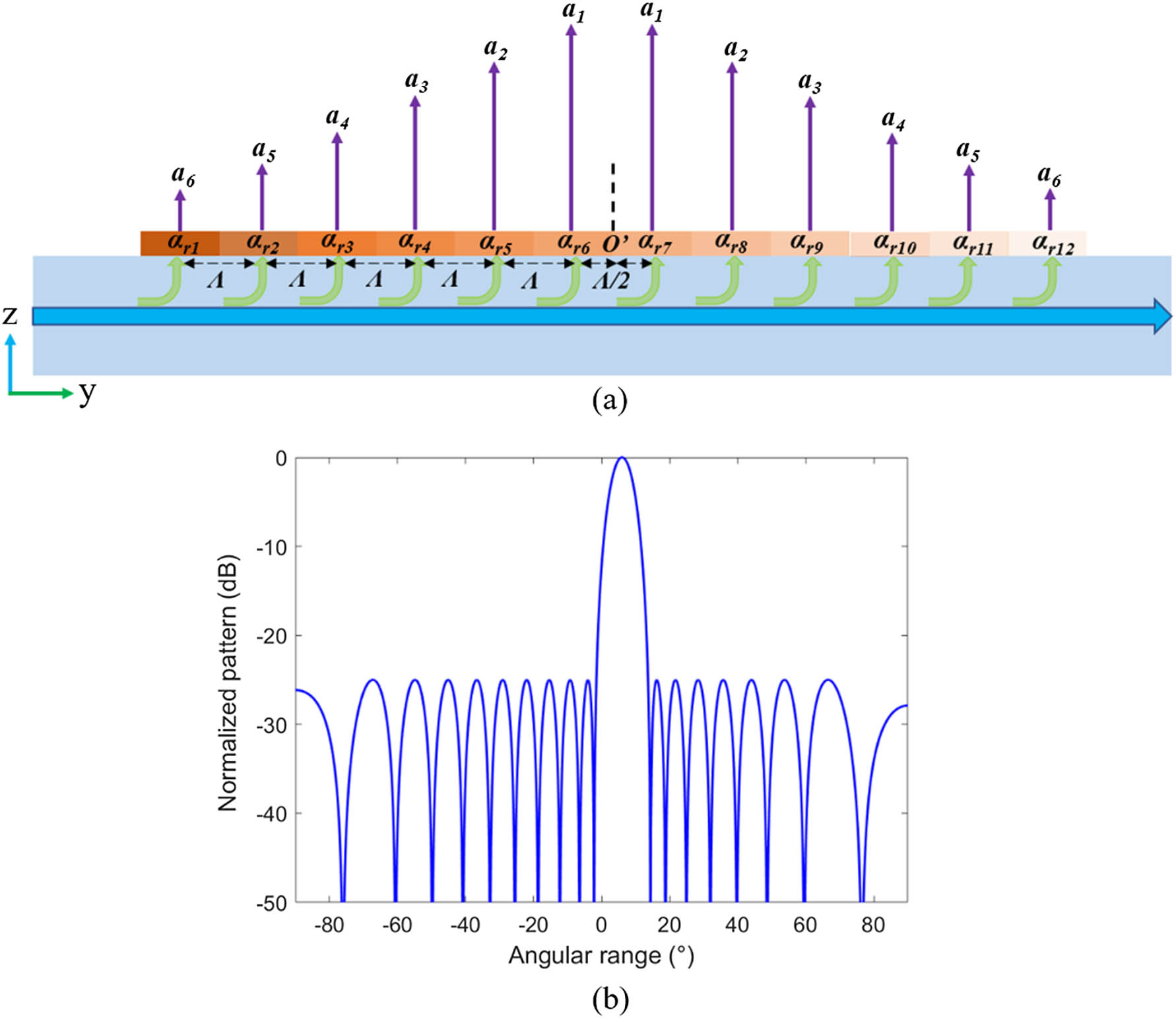#### Fig. 7. (a) Schematic diagram of a uniform linear array. (b) Radiation patterns in the xoz and yoz planes versus the number of antenna elements. (c) Array realized gain as a function of the number of antenna elements in the array.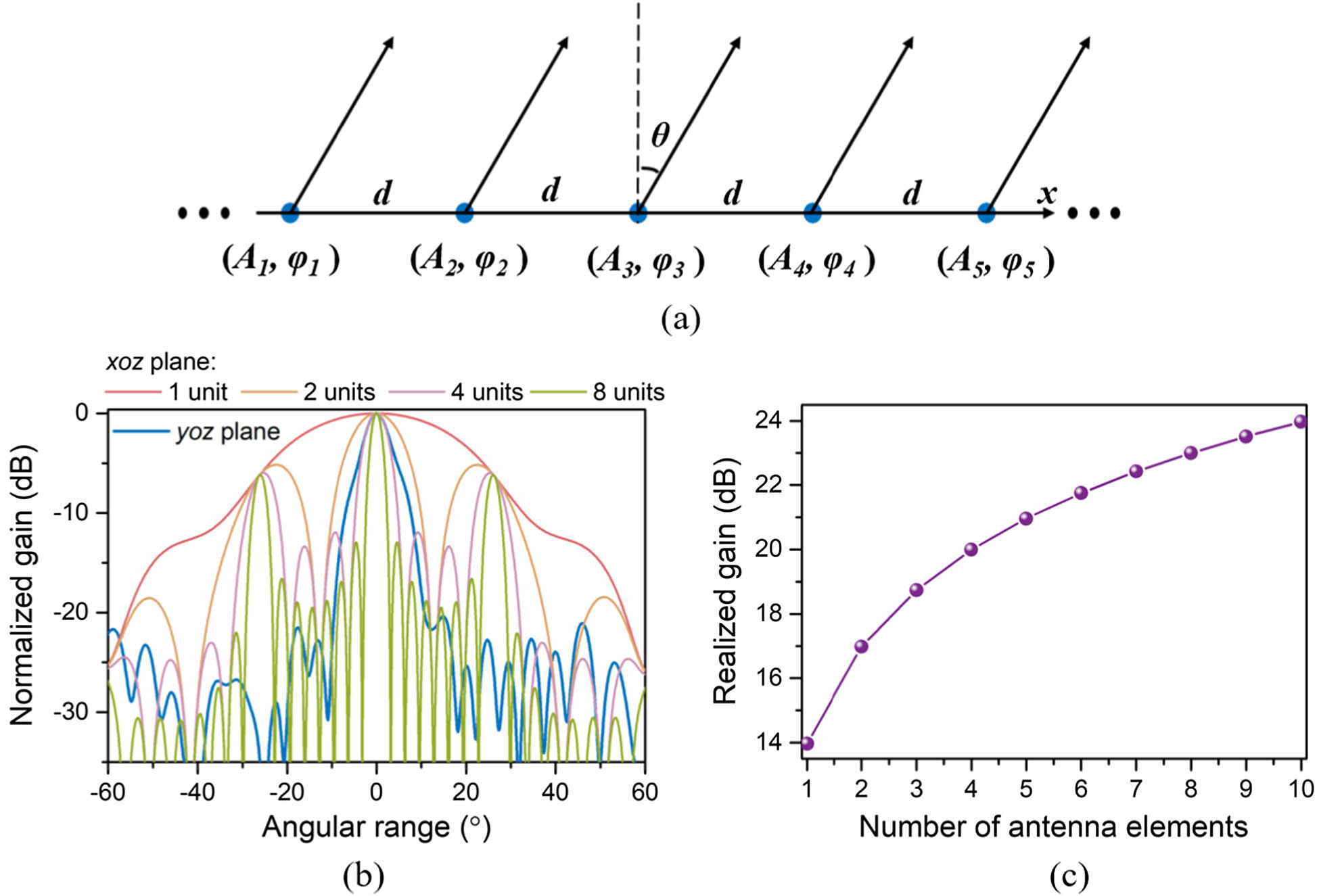Guang Zhu Zhou, Bao-Jie Chen, Geng-Bo Wu, Shi-Wei Qu, Chi Hou Chan. All-plasmonic optical leaky-wave antenna with a low sidelobe level[J]. Photonics Research, 2023, 11(9): 1500.